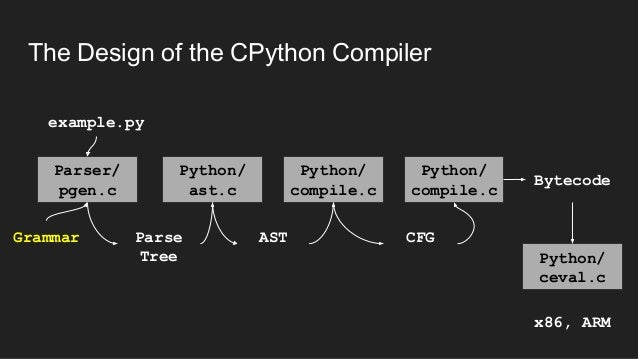# AST Parsing with Python to generate HP Calculator RPN

Posted by admin on September 22, 2018

How I used the Python AST capabilities to build the Python to Rpn converter.

My program works at the AST stage of the compilation pipeline:The converter does rely on the Python compiler. Specifically my approach has been to use Python's built in ability to parse itself into an AST Abstract Syntax Tree, then to traverse this tree using the visitor design pattern to generate the RPN.

For example, the following Python code:

Code:
`import astimport astunparseprint(astunparse.dump(ast.parse('x = 1 + 2')))`

will generate the following AST data structure representing x = 1 + 2:

Code:
`Module(body=[Assign(  targets=[Name(    id='x',    ctx=Store())],  value=BinOp(    left=Num(n=1),    op=Add(),    right=Num(n=2)))])`

If you are interested, here are the slides of a recent talk I gave to a local Python User Group in Melbourne, Australia, about how I used the Python AST capabilities to build the Python to Rpn converter. The reception to the talk was good, but became fun and buoyant once the audience realised I had targeted an old HP calculator with this fancy Python technology - especially when I pulled out my HP calcs for all to see and touch.

-Andy Bulka